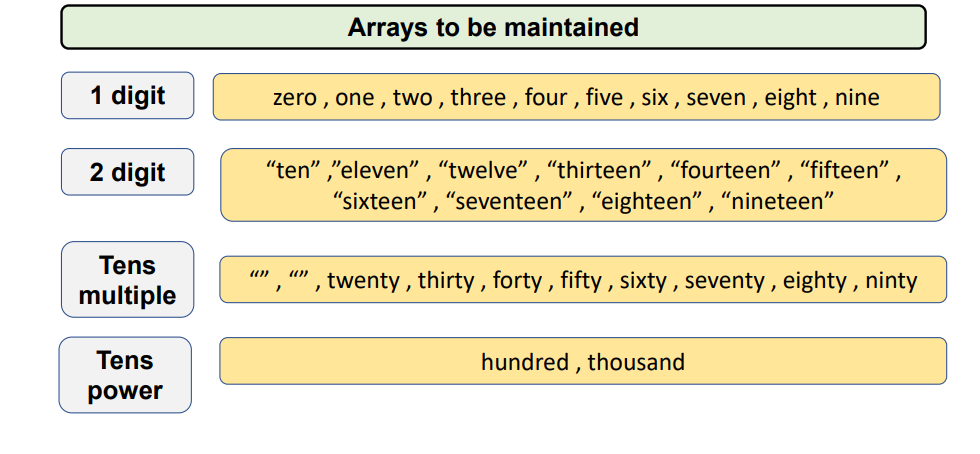# Convert digits/numbers to words

Problem Statement: Given a number, convert it into the form of words.

Note:- Consider maximum no. of digits in the number as 4.

Examples:

```Example 1:
Input: 7824
Output: seven thousand eight hundred twenty four
Explanation: 7824 in words can be written as seven thousand eight hundred twenty four.

Example 2:
Input: 370
Output: three hundred seventy
Explanation: 370 in words can be written as three hundred seventy.```

### Solution

Disclaimer: Don’t jump directly to the solution, try it out yourself first.

Approach

Here, we maintain some arrays of strings and use them for handling different cases.Case 1:  When a single digit is present

Case 2: When two digits are present

Subcases for 2 digits will be

-> having tens place as 1

-> having tens place as any other number other than 1 and 0

Case 3: When three digits are present

Just add hundred to hundredth place number, the further process will remain same as 2 digit number

Case 4: When 4 digits are present

Just add thousand to thousand place and further process will remain same as 3 digit number.

Code:

## C++ Code

``````#include <bits/stdc++.h>
using namespace std;

void convertnumintoword(string str)
{
string singledigit[] = {"zero", "one", "two", "three", "four", "five", "six", "seven", "eight", "nine"};
string twodigits[] = {"ten", "eleven", "twelve", "thirteen", "fourteen", "fifteen", "sixteen", "seventeen", "eighteen", "ninteen", "twelve"};
string tensmultiple[] = {"", "", "twenty", "thirty", "forty", "fifty", "sixty", "seventy", "eighty", "ninty"};
string tenspower[] = {"hundred", "thousand"};
if (str.length() == 0)
{
cout << "" << endl;
return;
}
else if (str.length() == 1)
{
cout << singledigit[str - '0'] << endl;
return;
}
int len = str.length();
for (int i = 0; i < str.length(); i++)
{
if (len > 2)
{
if (str[i] - '0' != 0)
{
cout << singledigit[str[i] - '0'] << " ";
cout << tenspower[len - 3] << " ";
}
len--;
}
else
{
if (str[i] - '0' == 1)
{
cout << twodigits[str[i + 1] - '0'] << " ";
return;
}
else if (str[i] - '0' != 0)
{
cout << tensmultiple[str[i] - '0'] << " ";
if (str[i + 1] - '0' != 0)
cout << singledigit[str[i + 1] - '0'] << " ";
return;
}
}
}
}

int main()
{
string str = "9090";
convertnumintoword(str);
return 0;
}
``````

Output: nine thousand ninty

Time Complexity: O(N) which is the length of string

Space Complexity: O(1)

## Java Code

``````import java.util.*;
public class Main {
public static void convertnumintoword(String str)
{
String []singledigit = new String[]{"zero", "one", "two", "three", "four", "five", "six", "seven", "eight", "nine"};
String []twodigits=new String[] {"ten", "eleven", "twelve", "thirteen", "fourteen", "fifteen", "sixteen", "seventeen", "eighteen", "ninteen", "twelve"};
String []tensmultiple = new String[]{"", "", "twenty", "thirty", "forty", "fifty", "sixty", "seventy", "eighty", "ninty"};
String []tenspower =new String[] {"hundred", "thousand"};
if(str.length()==0)
{
System.out.println("");
return;
}
else if(str.length()==1)
{
System.out.println(str.charAt(0)-'0');
return;
}

int len=str.length();

for(int i=0;i<str.length();i++)
{
if(len>2)
{
if(str.charAt(i)-'0'!=0)
{
System.out.print(singledigit[str.charAt(i)-'0']+" ");
System.out.print(tenspower[len-3]+" ");
}
len--;
}
else
{
if(str.charAt(i)-'0'==1)
{
System.out.print(twodigits[str.charAt(i+1)-'0']+" ");
return;
}
else if(str.charAt(i)-'0'!=0)
{
System.out.print(tensmultiple[str.charAt(i)-'0']+" ");
if(str.charAt(i+1)-'0'!=0)
System.out.print(singledigit[str.charAt(i+1)-'0']);
return;
}
}
}
}

public static void main(String args[]) {
String str="9090";
convertnumintoword(str);
}
}
``````

Time Complexity: O(N) which is the length of string

Space Complexity: O(1)

Special thanks to Gurmeet Singh for contributing to this article on takeUforward. If you also wish to share your knowledge with the takeUforward fam, please check out this article